# Decibel

A circuit has an input power of 58 mW. Its output power is 6 mW. What is the loss in decibels?

Correct result:

x =  9.85 dB

#### Solution:

$x = -10\cdot \log \dfrac{ 6 }{ 58 } = 9.85 \ \text{dB}$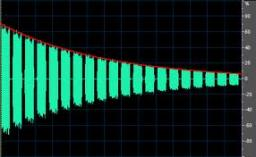We would be pleased if you find an error in the word problem, spelling mistakes, or inaccuracies and send it to us. Thank you!Tips to related online calculators
Check out our ratio calculator.

## Next similar math problems:

• Alopecia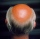Medical literature indicates that 45% of men suffer from alopecia. For random sample of 8 men, calculate the probability that: (a) exactly four men suffer from alopecia. (b) at most two men suffer from alopecia.
• Pascal's law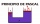Please calculate according to Pascal's law. Krupp's machines were known for their large size. In 1861, a blacksmith's steam hydraulic press was put into operation in Essen. What was the cross-sectional area of the larger piston if a compressive force of 1
• Assembly timeThe assembly time for the toy follows a normal distribution with a mean of 75 minutes and a standard deviation of 9 minutes. The company closes at 5 pm every day. If one starts assembling at 4 pm what is the probability that he will finish before the comp
• Up and down motionWe throw the body from a height h = 5 m above the Earth vertically upwards v0 = 10 m/s. How long before we have to let the second body fall freely from the same height to hit the Earth at the same time?
• DecibelBy what percentage does the sound intensity increase if the sound intensity level increases by 1 dB?
• Candles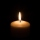Before Christmas, Eva bought two cylindrical candles - red and green. Red was 1 cm longer than green. She lit a red candle on Christmas Day at 5:30 p. M. , lit a green candle at 7:00 p. M. , and left them both on fire until they burned. At 9:30 p. M. , bo
• Inclined plane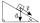1. How much work W we have to do to pull a body weighing 200 kg along an inclined plane with a length of 4 m to a total height of 1.5 m. 2. Find the force we need to exert to do this if we neglect frictional resistance. 3. Find the force we would need if
• Lowest voltage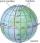Three resistors with resistors R1 = 10 kΩ, R2 = 20 kΩ, R3 = 30 kΩ are connected in series and an external voltage U = 30 V is connected to them. On which resistor is the lowest voltage?
• A particle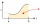A particle moves in a straight line so that its velocity (m/s) at time t seconds is given by v(t) = 3t2-4t-4, t>0. Initially the particle is 8 meters to the right of a fixed origin. After how many seconds is the particle at the origin?
• Self-oscillation period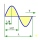The water in the vessel carried by the boy has a self-oscillation period of 0.8 s. What is the size of the boy's movement speed when the length of the boy's step is 60 cm? Give the result in m/s.
• Water current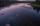John swims upstream. After a while, he passes the bottle, from that moment he floats for 20 minutes in the same direction. He then turns around and swims back, and from the first meeting with the bottle, he sails 2 kilometers before he reaches the bottle.
• Same force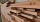The trunk of 5m length and 95 kilograms has a center of gravity 2m from the thicker end. The tribe is carried by two men, one at the thicker end. At what distance does the trunk carry a man from the other end to make the same force on it?
• Angled cyclist turnThe cyclist passes through a curve with a radius of 20 m at 25 km/h. How much angle does it have to bend from the vertical inward to the turn?
• Newtonmeters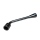The driver loosened the nut on the car wheel with a wrench that held 20 cm from the axis of the bolt. He acted on the key with a force of 320N. At what moment did he act on the bolt?
• Wheelchair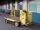The boy pushes the wheelchair with a force of F1 = 20N. The wheelchair exerts a force of F2 on the boy. How large is this force?
• Voltmeter range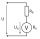We have a voltmeter which in the original set measures voltage to 10V. Calculate the size of the ballast resistor for this voltmeter, if we want to measure the voltage up to 50V. Voltmeter's internal resistance is 2 kiloohm/Volt.
• Accelerated motion - mechanicsThe delivery truck with a total weight of 3.6 t accelerates from 76km/h to 130km/h in the 0.286 km long way. How much was the force needed to achieve this acceleration?